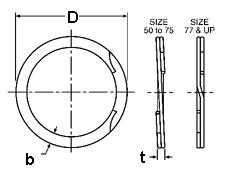### RRN-281

Display Units: inches |  metric
Request Quick Quote for this part.
 Ring Specs (D) Free Diameter: 3.001 +0.030 / -0.000 in. (t) Thickness: 0.093 +0.003 / -0.003 in. (b) Radial Wall: 0.225 +0.004 / -0.006 in. Groove Specs: (B) Application Diameter: 2.813 in. (G) Groove Diameter: 2.980 +0.006 / -0.006 in. (W) Groove Width: 0.103 +0.005 / -0.000 in. Groove Depth: 0.084 in. Other Specs Approximate Weight per 1000: (m) Material Thickness: 0.045 in. (N) Ring Number of Turns: 2.000 (Pg) Theoretical Thrust Load Capacity - Groove Yield:Notes: Yield Strength of Groove Material (Ys): 45,000 psi. Calculated using a safety factor (K) of 2Equation:Pg = [ B * d * Ys * pi ] / K    = [ (2.813 in.) * (0.084 in.) * (45,000 psi) * 3.14 ] / 2 16,694 lbs. (Pr) Theoretical Thrust Load Capacity - Ring Shear:Notes: Ring Material: Carbon Spring Steel (SAE 1070-1090). Shear Strength of Ring Material (Ss): 120,000 psi. Calculated using a safety factor (K) of 3Equation:Pr = [ B * t * Ss * pi ] / K    = [ (2.813 in.) * (0.093 in.) * (120,000 psi) * 3.14 ] / 3 32,858 lbs. Industry Equivalent Part Number(s):RRN-281, KG-281, WHM-281